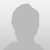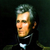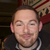+1

sovtek 7 years ago updated 7 years ago
Has anyone ever seen a complex .png crash an ipad? Not only does fuse box crash but the whole ipad restarts every time i view it.
``` <wire> <main> <panel name="panel" width="100%" height="100%" align="center" valign="center" background="#FFFFFF" draggable="yes" zoomable="yes"> <image name="background" source="FromFred/TextText_208ci22_1-22.png" width="100%" height="100%"></image> </panel> </main> </wire> ```I have not seen/experienced this. What kind of .png is it? Could you provide a link so we could grab it or view it?``` https://prod-web1.virpublisher.com/Vortex/sma/tmpFiles/text.png ```
The concept here is to render text over background images.i lowered the resolution down to 300 and it stopped crashing.This is than likely happening because of the size of the image in pixels. Are you intending to use such a large image for scrolling reasons? If not resize your image to fit the tablet/phone screen you are designing for.Hey Ian, this may be somewhat related to this thread. I have a JSON feed of around 90 items. Each item is a "page" that has information, including an image source. I am using to parse out each page with its preview image and page number.

If i try to list out all 96 images within a scrollable list, fusebox crashes on open. But if I chop it down to around 30, it seems to work fine. Each page image is 1024 x 1383.JPG 144dpiThe amount and size of the images could be affecting memory depending on the device you are looking at it on.

Are you loading the datasource oninit or based on an action? Have you considered using a tableview instead of a list?Ill try both, thanks for the response.Hey Ian.

I am now loading the datasource through an action when the panel is called to appear. But still, if I try to load all 96 items from the datasource (which is a list of thumbnails), the app crashes. When I bump it down to 35 items within my json feed, it works fine. So now, I am attempting to use a tableview instead of list, so that only the visible items are loaded. Problem is, my items don't seem to be appearing when using tableview. Could you tell me if you notice anything wrong with my tableview code compared to my list code. The list code works fine with only 35 items, but the tableview output never appears.

``````

<list name="pageList" width="100%" height="100%" datasource="pagesFeed" orientation="horizontal" paginate="no">

</object>

</list>

``````

``````

<tableview name="pageList" width="100%" rowheight="365" datasource="pagesFeed" orientation="horizontal" pagination="no" startindex="1">

</object>

</tableview>

``````Without seeing the complete code, it is hard to say. Your list is defining a height that the tableview is not.

Is your tableview displaying a scroll or is it not appearing at all?Its not appearing at all.This may help.

``````

<wire>

<datasources>

<datasource name="issueFeed" source="http://www.blanchardsystems.com/issue.json" query="/issue/node" providertype="json"/>

<datasource name="storiesFeed" source="http://www.blanchardsystems.com/stories.json" query="/stories/node" providertype="json" />

<datasource name="pagesFeed" source="" query="/pages/node" providertype="json" />

</datasources>

<styles>

<style name="bgRed" background="#ff0000" />

<style name="bgTan" background="#FFFFCC" />

<style name="bgGreen" background="#7B7922" />

<style name="bgOrange" background="#FF3300" />

</styles>

<classes>

<!-- Scrubber Page Class -->

<class name="pageItem">

<!--<image name="pageImg" width="226" height="305" source="[param:imgSrc]" onclick="page" clicktarget="../../../../../MAG" _page="[param:page]"></image>-->

<image name="pageImg" width="226" height="305" source="[param:imgSrc]" onclickup="showPageInfo" _storyId="[param:storyId]"></image>

<text name="pageNum" width="25" height="25" alignment="center" align="right" valign="bottom" font="helvetica" size="11" color="#ffffff" text="[param:id]"></text>

</panel>

</class>

<!-- Story Info Class -->

<!-- Give the class an alpha of 0. Then, when a certain toc is clicked (which should have the same story id), give that unique story info alias an alpha of 1 to make it appear -->

<class name="storyItem">

<panel name="storyInfoPanel-[param:id]" alias="STORYINFOPANEL-[param:id]" height="300" width="100%" valign="top">

<text name="storyType" width="70%" height="25" ypos="65" xpos="15" leftinset="15" color="#7c7c7c" font="Georgia" size="24%" align="left" text="[param:storyType]"></text>

<text name="storyName" width="70%" height="45" ypos="95" xpos="15" leftinset="15" color="#ffffff" font="Georgia" size="40%" align="left" text="[param:storyName]"></text>

<text name="storyAuthor" width="70%" height="15" ypos="140" xpos="15" leftinset="15" color="#7c7c7c" font="Georgia" size="13%" align="left" text="[param:storyAuthor]"></text>

<image name="scrubHr" widesource="ui/scrub_hrwide.png" ypos="160" xpos="15"></image>

<text name="storyInfo" width="70%" height="85" ypos="170" xpos="15" leftinset="15" color="#ffffff" font="Helvetica" size="13" align="left" text="[param:storyInfo]"></text>

<text name="storyTags" width="10%" height="25" ypos="140" color="#ffffff" font="Helvetica" size="13" align="right" text="Tags"></text>

<image name="storyPgNumBg" source="ui/scrub_pagenumbg.png" ypos="217" xpos="679" lxpos="909"></image>

<text name="storyNum" width="10%" height="25" ypos="217" color="#ffffff" font="Helvetica" size="13" align="right" text="[param:storyPage]"></text>

</panel>

</class>

<!-- TOC Item Class -->

<class name="tocItem">

<image name="tocItemBg" source="ui/toc_itembg.png" width="100%" height="100%"></image>

<image name="storyImage" source="[param:storyImage]" width="144" height="155" xpos="5" ypos="5" onclickup="hideToc" onclick="page" clicktarget="../../MAG" _page="[param:storyPage]"></image>

<text name="storyName" text="[param:storyName]" lwidth="750" width="530" height="30" alignment="left" xpos="170" ypos="15" font="Helvetica-Bold" size="19" color="#FFFFFF" onclickup="hideToc" onclick="page" clicktarget="../../MAG" _page="[param:storyPage]"></text>

<text name="storyInfo" lwidth="750" width="530" height="100" alignment="left" lypos="15" xpos="170" ypos="25" font="Helvetica" size="12" color="#BCBCBC" text="[param:storyInfo]"></text>

<text name="storyAuthor" lwidth="290" width="180" height="25" alignment="left" xpos="170" ypos="130" font="Helvetica-Oblique" size="12" color="#FFFFFF" text="by [param:storyAuthor]"></text>

<text name="storyPage" width="70" height="25" alignment="left" align="right" ypos="130" font="Helvetica-Bold" size="12" color="#FFFFFF" text="Page [param:storyPage]"></text>

</panel>

</class>

<!-- Old Double Column TOC Item Class

<class name="tocItemPanel">

<panel name="[param:name]" lwidth="462" width="350" height="170" ypos="[param:panelYpos]" xpos="[param:panelXpos]" lxpos="[param:panelLXpos]" onclickup="hideToc" onclick="page" clicktarget="../../MAG" _page="[param:_page]">

<image name="tocItemBg" source="ui/toc_itembg.png" width="100%" height="100%"></image>

<image name="[param:imgName]" source="[param:imgSource]" width="144" height="155" xpos="5" ypos="5"></image>

<text name="[param:titleName]" text="[param:titleText]" lwidth="290" width="180" height="30" align="right" alignment="left" ypos="5" font="Helvetica-Bold" size="17" color="#FFFFFF"></text>

<text name="[param:summaryName]" lwidth="290" width="180" height="120" align="right" alignment="left" lypos="5" ypos="15" font="Helvetica" size="12" color="#FFFFFF" text="[param:summaryText]"></text>

<text name="[param:authorName]" lwidth="290" width="180" height="25" align="right" alignment="left" ypos="140" font="Helvetica-Oblique" size="12" color="#FFFFFF" text="[param:authorText]"></text>

</panel>

</class>-->

</classes>

<actions>

<!-- On Init Action -->

<action name="oninit" oninit="yes">

<play action="setIssueName" />

</action>

<action name="setIssueName" datasource="issueFeed">

<assign property="object:ISSUENAME.text" value="[datasource:issueFeed.1.name]" />

</action>

<!-- Show page info in scrubber -->

<action name="showPageInfo" datasource="pagesFeed">

<sync>

<assign property="var:storyId" value="_storyId" />

<assign property="object:STORYTYPE.text" value="[datasource:storiesFeed.[var:storyId].type]" />

<assign property="object:STORYNAME.text" value="[datasource:storiesFeed.[var:storyId].name]" />

<assign property="object:STORYAUTHOR.text" value="by [datasource:storiesFeed.[var:storyId].author]" />

<assign property="object:STORYINFO.text" value="[datasource:storiesFeed.[var:storyId].info]" />

<assign property="object:STORYPAGE.text" value="Page [datasource:storiesFeed.[var:storyId].page]" />

<assign property="object:STORYTAGS.text" value="[datasource:storiesFeed.[var:storyId].tags]" />

</sync>

</action>

<!-- Toolbar Hide/Show -->

<action name="showNav">

<alpha target="MAINTOOLBAR" value=".95" time=".3"/>

<alpha target="TBSHDW" value="1" time=".3"/>

<set onclickup="hideNav" />

<!-- <sync delay="5">

<play action="hideNav">

</sync>	-->

</action>

<action name="hideNav">

<alpha value="0" target="MAINTOOLBAR" time="0"/>

<alpha target="TBSHDW" value="0" time=".3"/>

<set onclickup="showNav" />

</action>

<!-- Toolbar Home Button -->

<action name="homeBtnClick">

<!-- Put Home Button Action Code Here -->

</action>

<action name="homeBtnDown">

<!-- Show Home -->

<alpha value="1" target="HOMEBTNDN" time="0"/>

<alpha value="0" target="HOMEBTN" time="0"/>

<alpha value="0" target="BACKBTNDN" time="0"/>

<alpha value="0" target="TOCBTNDN" time="0"/>

<alpha value="0" target="SCRUBBTNDN" time="0"/>

<alpha value="0" target="SHAREBTNDN" time="0"/>

<alpha value="1" target="BACKBTN" time="0"/>

<alpha value="1" target="TOCBTN" time="0"/>

<alpha value="1" target="SCRUBBTN" time="0"/>

<alpha value="1" target="SHAREBTN" time="0"/>

</action>

<action name="homeBtnUp">

<alpha value="1" target="HOMEBTN" time="0"/>

<alpha value="0" target="HOMEBTNDN" time="0"/>

</action>

<!-- Toolbar Back Button -->

<action name="backBtnClick">

<!-- Put Back Button Action Code Here -->

<alpha value="1" target="BACKBTN" time="0"/>

<alpha value="0" target="BACKBTNDN" time="0"/>

</action>

<action name="backBtnDown">

<alpha value="0" target="BACKBTN" time="0"/>

<alpha value="1" target="BACKBTNDN" time="0"/>

<alpha value="0" target="HOMEBTNDN" time="0"/>

<alpha value="0" target="TOCBTNDN" time="0"/>

<alpha value="0" target="SCRUBBTNDN" time="0"/>

<alpha value="0" target="SHAREBTNDN" time="0"/>

<alpha value="1" target="HOMEBTN" time="0"/>

<alpha value="1" target="TOCBTN" time="0"/>

<alpha value="1" target="SCRUBBTN" time="0"/>

<alpha value="1" target="SHAREBTN" time="0"/>

</action>

<action name="backBtnUp">

<alpha value="1" target="BACKBTN" time="0"/>

<alpha value="0" target="BACKBTNDN" time="0"/>

</action>

<!-- Toolbar TOC Button -->

<action name="showToc">

<translate valign="top" time=".5" target="TOCPANEL"/>

<play action="tocBtnDown"/>

<!-- Make sure everything else is closed -->

<play action="hideScrubber"/>

<play action="hideShare"/>

</action>

<action name="hideToc">

<translate ypos="-101%" time=".5" target="TOCPANEL"/>

<play action="tocBtnUp"/>

<set onclickup="showToc" target="TOCBTN"/>

</action>

<action name="tocBtnDown">

<set onclickup="hideToc" target="TOCBTNDN"/>

<alpha value="0" target="TOCBTN" time="0"/>

<alpha value="1" target="TOCBTNDN" time="0"/>

</action>

<action name="tocBtnUp">

<alpha value="1" target="TOCBTN" time="0"/>

<alpha value="0" target="TOCBTNDN" time="0"/>

</action>

<!-- App Scrubber Actions -->

<action name="showScrubber">

<sync>

<alpha value="1" target="waiter"/>

<alpha value="0" target="pagePanel"/>

<alpha value="0" target="waiter"/>

<alpha value="1" target="pagePanel" time=".25"/>

</sync>

<!-- move whole panel to top -->

<translate valign="top" time=".5" target="SCRUBPANEL"/>

<play action="scrubBtnDown"/>

<play action="hideShare"/>

<play action="hideToc"/>

<assign property="datasource:pagesFeed.source" value="http://www.blanchardsystems.com/pages.json" />

</action>

<action name="hideScrubber">

<sync>

<alpha value="0" target="waiter"/>

<alpha value="1" target="pagePanel"/>

<alpha value="1" target="waiter"/>

<alpha value="0" target="pagePanel" time=".25"/>

</sync>

<translate ypos="-100%" time=".5" target="SCRUBPANEL"/>

<play action="scrubBtnUp"/>

<set onclickup="showScrubber" target="SCRUBBTN"/>

<assign property="datasource:pagesFeed.query"  value="/nothing" />

</action>

<action name="scrubBtnDown">

<set onclickup="hideScrubber" target="SCRUBBTNDN"/>

<alpha value="0" target="SCRUBBTN" time="0"/>

<alpha value="1" target="SCRUBBTNDN" time="0"/>

</action>

<action name="scrubBtnUp">

<alpha value="1" target="SCRUBBTN" time="0"/>

<alpha value="0" target="SCRUBBTNDN" time="0"/>

</action>

<!-- App Share Actions -->

<action name="showShare">

<translate align="right" time=".5" target="SHAREPANEL"/>

<play action="shareBtnDown"/>

<!-- Make sure everything else is closed -->

<play action="hideScrubber"/>

<play action="hideToc"/>

</action>

<action name="hideShare">

<translate xpos="100%" time=".5" target="SHAREPANEL"/>

<play action="shareBtnUp"/>

<set onclickup="showShare" target="SHAREBTN"/>

</action>

<action name="shareBtnDown">

<set onclickup="hideShare" target="SHAREBTNDN"/>

<alpha value="0" target="SHAREBTN" time="0"/>

<alpha value="1" target="SHAREBTNDN" time="0"/>

</action>

<action name="shareBtnUp">

<alpha value="1" target="SHAREBTN" time="0"/>

<alpha value="0" target="SHAREBTNDN" time="0"/>

</action>

<!-- ANIMATIONS -->

<anim name="page">

<scroll page="_page"/>

</anim>

<!-- Share panel -->

<!-- josh - make this work with our share panel -->

<anim name="share">

<alpha value="0"/>

<scale width="1%" height="1%" align="center" valign="center"/>

<scale width="90%" height="20%" time=".75" align="center" valign="center"/>

<alpha value="1" time="1"/>

<set _emailurl="_emailurl" _emailsubject="_emailsubject" target="buttons/email-panel"/>

</anim>

<anim name="close-share">

<scale width="1%" height="1%" valign="center" align="center" time=".75"/>

<alpha value="0" time=".75"/>

</anim>

<!-- email -->

<anim name="emailattach">

</anim>

<anim name="email">

</anim>

<!-- pdf -->

<!-- josh - can we use this? -->

<anim name="show-pdf">

<translate ypos="0" time="1" target="pdf" />

<sync loop="no" startdelay="0">

<alpha value="1" time="1" target="pdf/wait"/>

<alpha value="0" time=".5" target="pdf/wait" />

</sync>

</anim>

<anim name="hide-pdf">

<translate ypos="100%" time="1" target="pdf"/>

<sync loop="no" startdelay="0">

<alpha value="1" target="pdf/wait"/>

<alpha value="0" time=".5" target="pdf/wait"/>

</sync>

</anim>

</actions>

<main>

<!-- Blackout the bg -->

<panel name="background" width="100%" height="100%" background="#000000"></panel>

<panel alias="BODYCOLUMN" name="BODYCOLUMN" width="100%" height="100%">

<!-- Magazine -->

<panel alias="MAINCOLUMN" name="MNC" width="100%" height="100%" xpos="0" ypos="0" >

<!-- <include name="issue" alias="INCLUDE" height="100%" width="100%" align="left" valign="top"></include> -->

<pdf name="mag" alias="MAG" onclickup="showNav" source="issue/WITC.pdf" height="100%" width="100%" align="left" valign="top" spreads="yes" covertile="no">

</pdf>

</panel>

</panel>

<!-- Curtain: Used to highlight select component -->

<panel name="curtain" height="100%" width="100%" background="#0e0729" alpha="0"></panel>

<!-- SCRUBBER Offscreen-->

<panel name="SCRUBPANEL" alias="SCRUBPANEL" width="100%" height="100%" ypos="-100%" hidden="no">

<image name="scrubBottomBg" widesource="ui/scrub_bottombgwide.png" source="ui/scrub_bottombg.png" width="100%" ypos="282"></image>

<image name="scrubTopBg" source="ui/scrubbgtop.png" width="100%" xpos="0" height="300" ypos="0"></image>

<wait name="waiter" width="100%" height="100%"></wait>

<panel name="pagePanel" height="100%" width="100%" valign="bottom">

<!-- Story Info Panel -->

<panel name="storyInfoPanel" alias="STORYINFOPANEL" height="300" width="100%" valign="top">

<text name="storyType" alias="STORYTYPE" width="70%" height="25" ypos="65" xpos="15" leftinset="15" color="#7c7c7c" font="Georgia" size="24%" align="left" text=""></text>

<text name="storyName" alias="STORYNAME" width="70%" height="45" ypos="95" xpos="15" leftinset="15" color="#ffffff" font="Georgia" size="40%" align="left" text=""></text>

<text name="storyAuthor" alias="STORYAUTHOR" width="70%" height="15" ypos="140" xpos="15" leftinset="15" color="#7c7c7c" font="Georgia" size="13%" align="left" text=""></text>

<image name="scrubHr" source="ui/scrub_hr.png" widesource="ui/scrub_hrwide.png" width="525" height="2" lwidth="750" lheight="2" ypos="160" xpos="15"></image>

<text name="storyInfo" alias="STORYINFO" width="70%" height="85" ypos="170" xpos="15" leftinset="15" color="#ffffff" font="Helvetica" size="13" align="left" text=""></text>

<text name="storyTagsLabel" alias="STORYTAGSLABEL" width="80" height="20" ypos="140" xpos="660" lxpos="910" color="#ffffff" alignment="right" font="Helvetica" size="13" text="Story Tags:"></text>

<text name="storyTags" alias="STORYTAGS" width="180" lwidth="190" height="55" ypos="155" xpos="558" lxpos="790" alignment="right" color="#ffffff" font="Helvetica" size="13" text=""></text>

<image name="storyPgNumBg" source="ui/scrub_pagenumbg.png" ypos="225" xpos="679" lxpos="919"></image>

<text name="storyPage" alias="STORYPAGE" width="10%" height="25" color="#ffffff" font="Helvetica" size="13" ypos="225" xpos="689" lxpos="929" text=""></text>

</panel>

<!-- Scrubber Horizontal Page Listing -->

<panel name="pageListing" width="100%" height="365" lheight="440" lypos="315" ypos="315">

<pager width="100%" height="365" lheight="440" name="contents" align="center" hidden="no" paginate="yes">

<list name="pageList" width="100%" height="100%" datasource="pagesFeed" orientation="horizontal" paginate="no">

</list>

<!--

<tableview name="pageList" width="100%" rowheight="365" datasource="pagesFeed" orientation="horizontal" pagination="no" startindex="1">

</tableview>

-->

</pager>

</panel>

<panel name="highlight-white" background="#ffffff" alpha=".1" width="100%" height="1" valign="top"></panel>

<panel name="highlight-black" background="#000000" alpha=".5" width="100%" height="1" topof="highlight-white"></panel>

</panel><!-- end pagePanel -->

</panel><!-- end SCRUBPANEL -->

<!-- APP SHARE PANEL (offscreen)-->

<panel name="SHAREPANEL" alias="SHAREPANEL" width="304" height="100%" xpos="100%" hidden="no" background="#000000">

<image name="shareBg" source="ui/share_bg.png" height="100%" width="100%"></image>

<panel name="actionsPanel" alias="ACTIONSPANEL" width="304" height="400" ypos="43">

<image name="shareTitleBg" source="ui/share_titlebg.png" height="41" width="100%"></image>

<text name="shareActions" width="276" height="39" align="right" size="17" font="helvetica" text="Actions" color="#FFFFFF" ypos="2"></text>

<image name="fbIcon" source="ui/share_fb.png" height="82" width="82" xpos="112" ypos="50"></image>

<text name="fbTxt" width="82" height="25" alignment="center" size="13" font="helvetica" text="Facebook" color="#FFFFFF" xpos="112" ypos="137"></text>

<image name="emailIcon" source="ui/share_email.png" height="82" width="82" xpos="210" ypos="50"></image>

<text name="emailTxt" width="82" height="25" alignment="center" size="13" font="helvetica" text="Email" color="#FFFFFF" xpos="210" ypos="137"></text>

<image name="copyIcon" source="ui/share_copy.png" height="82" width="82" xpos="15" ypos="165"></image>

<text name="copyTxt" width="82" height="25" alignment="center" size="13" font="helvetica" text="Copy Link" color="#FFFFFF" xpos="15" ypos="252"></text>

<image name="favIcon" source="ui/share_favorite.png" height="82" width="82" xpos="112" ypos="165"></image>

<text name="favTxt" width="82" height="25" alignment="center" size="13" font="helvetica" text="Add Favorite" color="#FFFFFF" xpos="112" ypos="252"></text>

</panel>

<panel name="favsPanel" alias="FAVPANEL" width="304" height="350" ypos="445">

<image name="favTitleBg" source="ui/share_titlebg.png" height="41" width="100%"></image>

<text name="favActions" width="276" height="39" align="right" size="17" font="helvetica" text="Favorites" color="#FFFFFF" ypos="2"></text>

</panel>

</panel>

<!-- END APP SHARE PANEL -->

<!-- APP TOC SCREEN offscreen-->

<panel name="TOCPANEL" alias="TOCPANEL" width="100%" height="100%" ypos="-105%" hidden="no" background="#000000" onclickup="showNav">

<!--<web name="WEBDATATEST" alias="WEBDATATEST" zoomable="no" file="tests/test.html" width="100%" height="100%"></web>-->

<image name="tocBg" source="ui/toc_bg.png" width="100%" height="100%"></image>

<list name="tocList" alias="TOCLIST" width="100%" height="100%" datasource="storiesFeed" orientation="vertical" paginate="no">

<object datasource="storiesFeed" name="tocItem" class="tocItem" id="[param:id]" size="12" storyName="[param:name]" storyPage="[param:page]" storyAuthor="[param:author]" storyInfo="[param:info]" storyType="[param:type]" storyImage="[param:image]"></object>

</list>

</panel>

<!-- APP TOOLBAR -->

<panel alias="MAINTOOLBAR" alpha="0" name="MAINTOOLBAR" width="100%" height="55" xpos="0" ypos="0">

<image name="TBBG" height="44" width="100%"  alpha=".95" xpos="0" ypos="0" source="ui/tbbg.png"></image>

<text name="issueName" alias="ISSUENAME" text="" width="400" height="100%" align="center" alignment="center" valign="top" font="Helvetica" size="17" color="#FFFFFF"></text>

<panel name="TBSUB" height="44" width="100%" xpos="0" ypos="0">

<panel name="LSPACE2" width="10" height="100%" align="left"></panel>

<panel name="LEFTBUTTONS" fitwidth="yes" height="100%" align="left" rightof="LSPACE2">

<image name="HOMEBTN" alias="HOMEBTN" onclick="homeBtnDown" onclickup="homeBtnClick" source="ui/home.png" height="44" valign="center" align="left"></image>

<image name="HOMEBTNDN" alias="HOMEBTNDN" source="ui/homehlb.png" alpha="0" height="44" valign="center" align="left"></image>

<image name="BACKBTN" alias="BACKBTN" source="ui/back.png"  height="44" onclick="backBtnDown" onclickup="backBtnClick" alpha="1"  valign="center" align="left" rightof="HOMEBTN"></image>

<image name="BACKBTNDN" alias="BACKBTNDN" source="ui/backhlb.png" height="44" onclickup="backBtnUp" alpha="0"  valign="center" align="left" rightof="HOMEBTN"></image>

<image name="TOCBTN" alias="TOCBTN" onclick="tocBtnDown" onclickup="showToc" source="ui/toc.png" height="44" valign="center" align="left" rightof="BACKBTN"></image>

<image name="TOCBTNDN" alias="TOCBTNDN" source="ui/tochlb.png" alpha="0" height="44" valign="center" align="left" rightof="BACKBTN"></image>

</panel>

<panel name="RSPACE1" width="10" height="100%" align="right"></panel>

<panel name="RIGHTBUTTONS" width="50%" height="100%" align="right" leftof="RSPACE1">

<image name="SHAREBTN" alias="SHAREBTN" onclickup="showShare" source="ui/share.png" height="44" valign="center" align="right"></image>

<image name="SHAREBTNDN" alias="SHAREBTNDN" source="ui/sharehlb.png" alpha="0" height="44" valign="center" align="right"></image>

<image name="SCRUBBTN" alias="SCRUBBTN" onclickup="showScrubber" source="ui/scrub.png" height="44" valign="center" align="right" leftof="SHAREBTN"></image>

<image name="SCRUBBTNDN" alias="SCRUBBTNDN" source="ui/scrubhlb.png" height="44" alpha="0" valign="center" align="right" leftof="SHAREBTN"></image>

</panel>

<panel alias="bb2LQHTZZ" name="bb2LQHTZZ" ypos="43" height="1" width="100%" background="#000000"></panel>

</panel>

<image name="TBSHDW" alias="TBSHDW" alpha="0" source="ui/tbdropshadow.png" height="11" width="100%" xpos="0" ypos="44"></image>

</panel>

</platform>

</main>

</wire>``````your tableview is using an attribute called "pagination", that should read "paginate"Ok, ill test to see if that was the problem. I was wondering why the two were listed as different attributes for list and tableview. You may want to edit this page: https://studio.rarewire.com/wordpress... . You have it listed as "pagination" for tableviewCode is now as follows:

``````

<tableview name="pageList" width="100%" rowheight="100%" datasource="pagesFeed" orientation="horizontal" paginate="no" startindex="1">

</tableview>

``````

Still doesn't work, althought list works fine.try giving your tableview a height.That worked! But it seems Im still running into the same issue when attempting to load more than 35 of my jSON feed thumbnail items. Nothing appears in the area.

Have their been issues with attempting to load this number of images before?

Is there a different method I should try in order to load a certain amount of images at once within this tableview?Ian, there may have been an issue with my feed I think. I will continue debugging. Thanks for your help on the tableview.We will also have to do this for large versions of all 96 pages. Do you think this will be a problem?